# GMAT Math : DSQ: Graphing a line

## Example Questions

### Example Question #1 : Dsq: Graphing A Line

Graph a line, if possible.

Statement 1: The slope is 4.

Statement 2: The y-intercept is 4.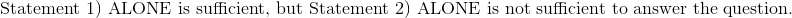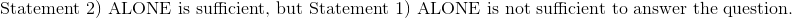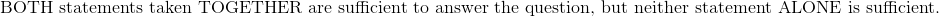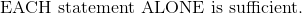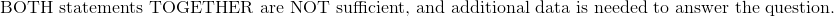Explanation:

Statement 1): The slope is 4.

Write the slope-intercept form, and substitute the slope.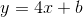The point and the y-intercept are unknown.  Either of these will be needed to solve for the graph of this line.

Statement 1) by itself is not sufficient to graph a line.

Statement 2): The y-intercept is 4.

Substitute the y-intercept into the incomplete formula.

The function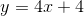can then be graphed on the x-y coordinate plane.

Therefore:### Example Question #2 : Dsq: Graphing A Line

Find the graph of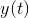.

I)is a linear equation which passes through the point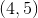.

II)crosses the y-axis at 1300.

Statement I is sufficient to answer the question, but Statement II is not sufficient to answer the question.

Either statement is sufficient to answer the question.

Both statements are needed to answer the question.

Statement II is sufficient to answer the question, but Statement I is not sufficient to answer the question.

Both statements are needed to answer the question.

Explanation:

Find the graph of.

I)is a linear equation which passes through the point.

II)crosses the y-axis at 1300.

To graph a linear equation, we need some combination of slope, y-intercept, or two points.

Statement I tells usis linear and gives us one point.

Statement II gives us the y-intercept of.

We can use Statement I and Statement II to find the slope of. Then, we can plot the given points and continue the line in either direction to get our graph.

Slope: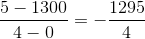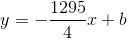Plugging in the provided value of, 1300, we have the equation of the line: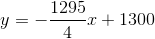Tired of practice problems?

Try live online GMAT Math prep today.